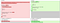# My First Try On TypeScript Programming

I have tried coding in JavaScript using different standard, start from ES5 to ES6. My impression was gained when I tried some simple code using ES6. Why? Because in ES6 I can declare class, even I know that when it translated to ES5 it just a variable which return a function.

For example:

`class MyClass {    greeting(name) {    return "Hello " + name;  }  }`

Which later will be translated to ES5 as below:

`"use strict";function _classCallCheck(instance, Constructor) { if (!(instance instanceof Constructor)) { throw new TypeError("Cannot call a class as a function"); } }var MyClass = function () {  function MyClass() {    _classCallCheck(this, MyClass);  }  MyClass.prototype.greeting = function greeting(name) {    return "Hello " + name;  };  return MyClass;}();`

For simple and non-dependent code (single file only), you can use BabelJS REPL. But, for real application, I suggest you to use Grunt or Gulp to make converter task from ES6 standard to ES5 using BabelJS.

Here is the gulpfile.js example to convert from ES6 to ES5 (ES2015):

`const gulp = require('gulp');const babel = require('gulp-babel');gulp.task('convert', () =>  gulp.src('src/**/*.js')  .pipe(babel({    presets: ['es2015']  }))  .pipe(gulp.dest('dist')));gulp.task('watch', ['convert'], () => {  gulp.watch('src/**/*.js', ['convert']);});gulp.task('default', ['watch'])`

Using above code, you can now write your JS code using ES6 standard. I will not show you the rest of other code, you can learn by your self and see ES6 features from this website:

I didn’t dive into TypeScript deeply yet, at first try I don’t see any differences between JS using ES6 standard, except TypeScript support static typing binding. Furthermore, I found TypeScript have controlling access to member class. This prevent you to access wrong variable value before it has assigned.

For instance, you have following code in ES6 standard (and later compiled using BabelJS):

`// Multiply.jsclass Multiply {    constructor(a, b) {    this.a = a;    this.b = b;  }    result() {    return this.a * this.b;  }  }let multiply = new Multiply(2, 3);console.log(multiply.a);console.log(multiply.b);console.log(multiply.result());`

and below code written using TypeScript:

`// Multiply.tsclass Multiply {  private a: number;  private b: number;    constructor(a: number, b: number) {    this.a = a;    this.b = b;  }    result() {    return this.a * this.b;  }  }let multiply = new Multiply(2, 3);console.log(multiply.a);console.log(multiply.b);console.log(multiply.result());`

You may noticed, that Multiply.js is just standard JS code and Multiply.ts is JS code + type checking + access level attribute. For the first one code, you can convert it into ES5 using BabelJS preset `es2015-loose`. For the second one, you can compile it using TypeScript with following configuration (tsconfig.json):

`{    "compilerOptions": {        "target": "es5",        "module": "commonjs",        "outDir": "dist",        "moduleResolution": "node",        "emitDecoratorMetadata": true,        "experimentalDecorators": true,        "sourceMap": true,        "alwaysStrict": true    },    "exclude": [        "node_modules"    ]}`

If you have expect that both code results same code after compiled into ES5, you’re right! Here is the diffs:Multiply.js and Multiply.ts after compiled into Multiply.js using ES5 preset. Left code is from Multiply.js and the right one is from Multipy.ts

There are no many differences between above two files, just class type-checking in BabelJS compile result.

The answer for this question is depends. If you see from the language (JavaScript) itself, it’s not a static typing languange inborn. But, “transpiler” like BabelJS or JavaScript superset like TypeScript, is just checking the type of variable and just return error notice, but don’t stop it from translates into JavaScript code.

However, if you see from TypeScript side, you may consider that TypeScript is a type-checking (okay we can call it “partial type-checking”), since it just check the type of your assigned variable parameter and doesn’t stop you to convert it as JavaScript ES5 code — it just complains you. This also occurred when you try to access private or protected member of a class.

I think TypeScript have its own future. It redefine JavaScript syntax into clearer and nicer syntax — oh I almost forgot about mentioned it as neat language compared to JavaScript. TypeScript will allow JS developer to code in logical syntax, no more`var ClassName = function () {}` each times they want to declare a class (although eventually it will be converted into it).